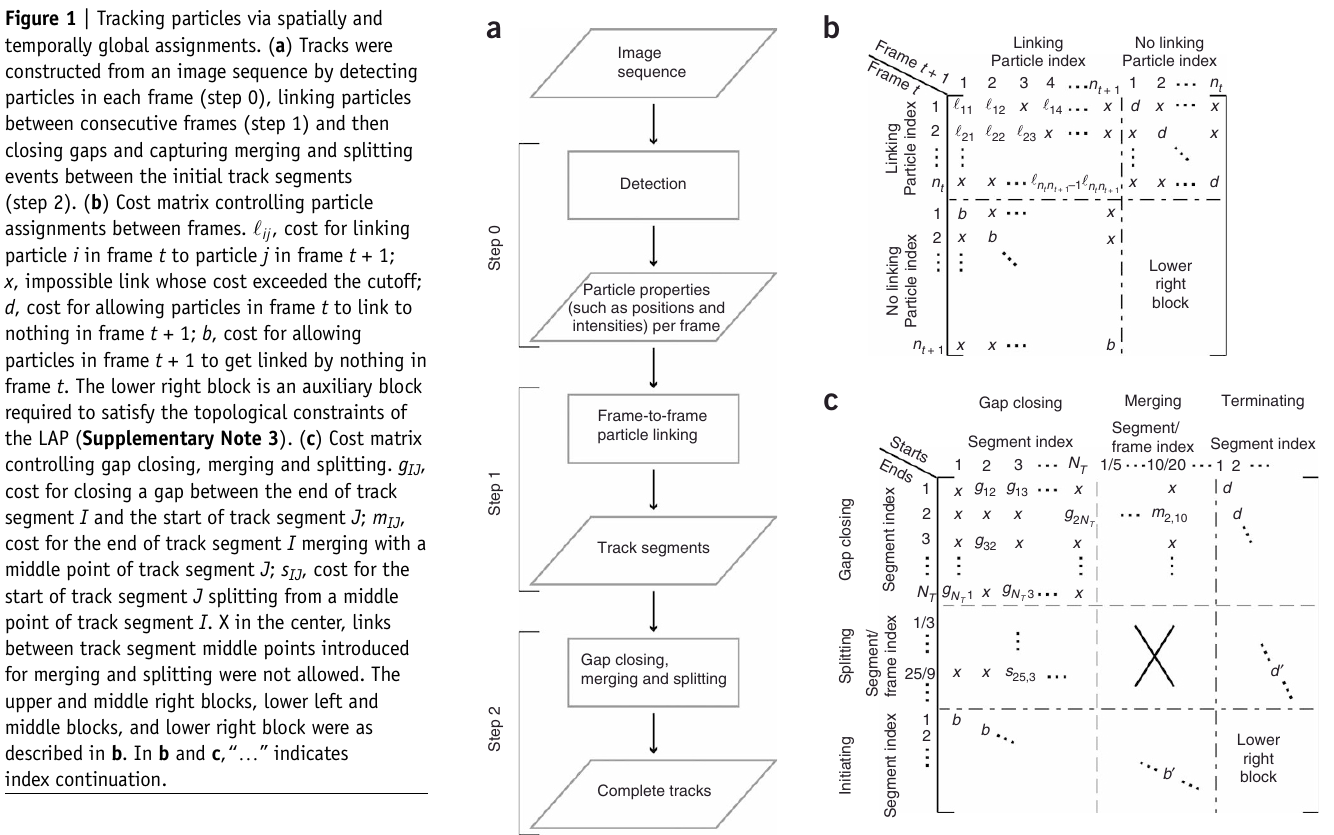# Particle Tracking as Linear Assignment Problem

##### Posted on Sep 24, 2020 (Update: Sep 28, 2020)

Present a tracking algorithm that addresses the principal challenge of Single-particle tracking (SPT), namely

• high particle density
• particle motion heterogeneity
• temporary particle disappearance
• particle merging and splitting

The algorithm first links particles between consecutive frames and then links the resulting track segments into complete trajectories. Both steps are formulated as global combinatorial optimization problems whose solution identifies the overall most likely set of particle trajectories throughout a movie.

SPT goes beyond the detection and localization of particles; its key step is the establishment of correspondence between particle images in a sequence of frames.

In multiple-hypothesis tracking (MHT), given particle positions in every frame, all particle paths within the bounds of expected particle behavior are constructed throughout an entire movie. The largest nonconflicting ensemble of paths is then chosen as the solution, and the solution is globally optimal in both space and time. But it is computationally prohibitive.

In the LAP framework, every potential assignment (partial assignment in the first step, track segment assignment in the second step) was characterized by a cost $C$. The goal of solving the LAP in each step was then to identify the combination of assignments with the minimal sum of costs:

$\hat A_{\arg\min} = \sum_{i=1}^{nrow}\sum_{j=1}^{ncol} A_{ij}C_{ij}\,,$

such that

$\sum_{i=1}^{nrow} A_{ij} = 1,\quad \sum_{j=1}^{ncol}A_{ij}=1\,.$

In the gap closing, merging and splitting step, six types of potential assignments were in competition.

• close a gap $g$: the end of a track segment could link to the start of another track segment
• merge $m$: the end of a track segment could link to a middle point of another track segment
• split $s$: the start of a track segment could get linked by a middle point of another track segment
• termination $d$: the end of a track segment could link to nothing
• initiation $b$: the start of a track segment could get linked by nothing
• refuse a merge or a split ($d’$ and $b’$): the track segment middle points introduced for merging and splitting could link to nothing.The framework is independent of problem dimensionality (2D or 3D) as well as of the type of particle motion (Brownian motion and directed motion, among others). It is also independent of the physical nature of the particle (single molecule, molecular assembly, organelle and others), which mainly influences the choice of an appropriate particle-detection method. But the cost function must be tailored to be specific tracking application.

Published in categories Note